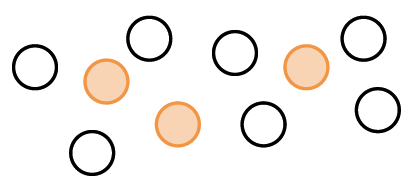Home > CC1MN > Chapter cc21 > Lesson cc21.2.6 > Problem1-116

1-116.

Mario was visiting the carnival when he noticed a few number relationships. He made them into brainteasers for you. Homework Help ✎

1. If three tenths of the visitors were adults and there were $100$ visitors, how many visitors were adults?If the shaded circles represent the fraction of adults, how many adults were present at the carnival?

Each circle is representative of $10$ visitors.

There were $30$ adults at the carnival.

2. Five eighths of the prizes at the Giant Spin were dolls. If there were $64$ prizes, how many prizes were not dolls?If the shaded boxes represent the fraction of prizes that were dolls, what fraction of the prizes were not dolls?

If there were $64$ total prizes, how many prizes does each square represent?